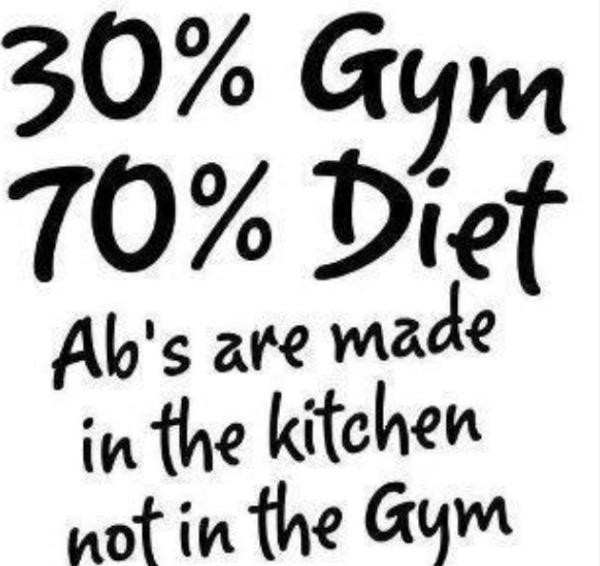# Maintaining Diet

## To figure out basics of the healthy balanced diet for the needs of your training## Remember exercising is easy the hardest part is to keep the diet.

###### Step 1:

Firstly we have to calculate the need of calories intake in your diet which is a fundamental stage of any diet as I will explain in further steps. To calculate calories it is the best to use 'Harris Benedict Equation' as it will estimate energy required to maintain your current weight without any exercise. After completing this step we can add our exercise level.

Equation

BMR calculation for men (metric)BMR = 66.5 + ( 13.75 x weight in kg ) + ( 5.003 x height in cm ) – ( 6.755 x age in years )

BMR calculation for men (imperial)BMR = 66 + ( 6.2 x weight in pounds ) + ( 12.7 x height in inches ) – ( 6.76 x age in years )

BMR calculation for women (metric)BMR = 655 + ( 9.563 x weight in kg ) + ( 1.850 x height in cm ) – ( 4.676 x age in years )

BMR calculation for women (imperial)BMR = 65.5 + ( 4.35 x weight in pounds ) + ( 4.7 x height in inches ) - ( 4.7 x age in years )

Example

Here is an example of how the equation works. Men of weight 100kg (220lb) and height 173cm (68inch). We have used 2nd equation in imperial measures but it can be calculated in metric as well.

100kg = 220 lb 173cm = 68 inches

66 + (6.23 x weight in pounds) + (12.7 x height in inches) – (6.8 x age in years)

1436.6 + 863.6 – 149.6 = 2150.6

###### Step 2:

Is the exercise level that we are at the moment.

Little to no exercise Daily kilocalories needed = BMR x 1.2

Light exercise (1–3 days per week)Daily kilocalories needed = BMR x 1.375

Moderate exercise (3–5 days per week)Daily kilocalories needed = BMR x 1.55

Heavy exercise (6–7 days per week)Daily kilocalories needed = BMR x 1.725

Very heavy exercise (twice per day, extra heavy workouts)Daily kilocalories needed = BMR x 1.9

So we add the exercise level to our example equation

1436.6 + 863.6 – 149.6 = 2150.6 x 1.55 = 3333 calories

To sum up of what we already we have checked how often the men exercises as he exercises 4 days a week the exercise level multiplier will be 1.55 and it will result in total calories he needs to maintain his diet.

###### Step 3:

To lose weight -500

To maintain it 0

To gain +250 to +300

To finish our equation for our example: 1436.6 + 863.6 – 149.6 = 2150.6 x 1.55 = 3333 calories – 500 = 2833 As the men wants to lose weight we will deduct 500 from the total value which shows result of how much calories he has to intake on daily basis.

###### Step 4:

We need to split our total calories into meals, the best was is to split it to 5 meals.

2833 / 5 meals = 566.6 calories per meal

566 calories - meal 1

366 calories – meal 2

666 calories – meal 3

366 calories – meal 4

666 calories - meal 5

However apart from just calories it is the best to calculate proteins, carbohydrates and fat intake to spread it between the meals which we will discuss in part 2.

This may seem hard or mathematical however it is really important to know how much calories we consume and how much we should intake daily. If you find it confusing check Muscle Mass Brothers Forum we can help you out with calculating your diet and help you with tips and trick of how to keep it healthy, fit and balanced.

We will post part 2 Basics of Diet where we will discuss carbohydrate, fat and proteins and their importance to diet.

No tags yet.